# Image Processing Programs

The programs are written to do a number of image processing tasks.

• ACTIVECONTOUR Active contour (or snake) example program. Uses GVF Active Contouring algorithm.
• cgASINHSCL Similar to BYTSCL, except that an inverse hyperbolic sine function is used for scaling.
• cgCLIPSCL Performs a linear byte scaling of data, with clipping on each end of the histogram.
• cgCOMPRESSSCL Compresses image mid-tones in a scaling transformation.
• cgGMASCL BYTSCL on steroids! Gamma as well as linear scaling.
• cgGAUSSSCL Performs a Gaussian scaling on image arrays.
• cgLOGSCL Like BYTSCL, only with a logrithmic scaling instead of linear scaling.
• cgSDEVSCL Like BYTSCL, but using the standard deviation of pixels to create scaling thresholds.
• cgSTRETCH Interactively increase image contrast with various image pixel transformations.
• CHECKERBOARD Creates a 2D checkerboard image.
• FIT_ELLIPSE Fits an ellipse to a region of interest (known as a "blob").
• HIST_ND Calculates an N-dimensional histogram.
• HISTOMATCH Performs histogram matching on an image.
• SCALEMODIS Provides differential scaling to brighten MODIS reflectance images.
• SHARPEN Sharpens an image with a Laplacian kernel.#### ACTIVECONTOUR

This is a demonstration program for experimenting with active contours or snakes. It uses the Gradient Vector Flow (GVF) method of active contouring by Chenyang Xu and Jerry Prince. For details of the method and pointers to articles and other information, see Active Contouring (Snakes) in IDL. This program can be purchased from the Coyote Store.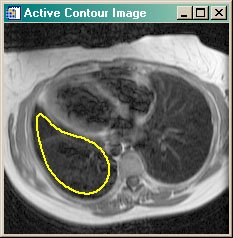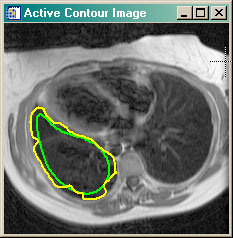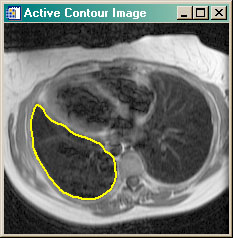#### cgASINHSCL

This is an image processing utility routine similar to cgGMASCL in that it scales input data into the range of 0 to 255. But it uses an inverse hyperbolic sine scaling algorithm, rather than a power-law (gamma) scaling algorithm. This is extremely useful when you have an image with a low signal to noise ratio. It can compress the noise, which simulataneously expanding the signal. You can specify both the minimum and maximun scaling output values, and you can obtain a negative image by simply setting the Negative keyword. The shape of the hyperbolic sine curve can be modified with a “softening” parameter, named beta. The input is scaled with the following equation before the final output is scaled into the range 0 to 255:
scaled = ASINH(beta*image)

Here is a picture of what the output looks like when the input is INDGEN(256). Although the output resembles a gamma function with the default beta parameter, the function can be “tuned” to work with even those very difficult FITS images. The cgSTRETCH program can be used as an interactive front end to this program.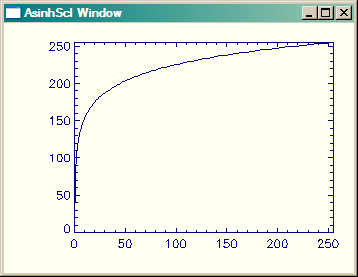#### cgCLIPSCL

This is an image processing utility routine similar to BYTSCL in that it scales input data into the range of 0 to 255. But it differs in that a user-specified percentage of pixels can be clipped from either end of the image histogram. The default it to use a two percent clip. (What ENVI calls a linear 2% stretch.) Clipping is approximate, and depends entirely on the image histogram. To clip 5 percent of the pixels from both ends of the image histogram before stretching, type this command. The cgSTRETCH program can be used as an interactive front end to this program.
```   IDL> cgImage, cgClipScl(image, 5)
```

#### cgCOMPRESSSCL

This is an image processing utility routine similar to GMASCL in that it scales input data into the range of 0 to 255. But it uses a compression scaling algorithm, rather than a linear or gamma (power-law) scaling algorithm. This is extremely useful when you want to compress information in the upper and lower data values, while stretching the area around a mean value. You can specify both the minimum and maximun scaling output values, and you can obtain a negative image by simply setting the Negative keyword. The center of the scaling curve can be set with a Mean keyword, and the slope of the curve can be set by selecting higher values of Exponent. The input is scaled with this equation before the final output is scaled into the range 0 to 255:
scaled = 1 / ( 1 + (mean/input)^exponent)

Here is a picture of what the output looks like when the input is INDGEN(256). The cgSTRETCH program can be used as an interactive front end to this program.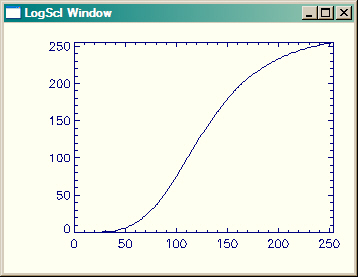#### cgGAUSSSCL

This is an image processing utility routine similar to cgGMASCL in that it scales input data into the range of 0 to 255. But it uses an Gaussian (normal) scaling algorithm, rather than a power-law (gamma) scaling algorithm. Interactive Gaussian stretching can be accomplished with cgSTRETCH.

Here is a picture of what the output looks like with various Gaussian values when the input is INDGEN(256).#### cgGMASCL

I think of this program as “BYTSCL on steroids!” It scales image data in the manner of BYTSCL, but it also allows gamma log scaling in addition to the standard linear scaling. Moreover, you can specify both the minimum and maximun scaling output values, and you can obtain a negative image by simply setting the Negative keyword. I wrote the program primarily to deal with gnarly FITS images with their often complex image contrast requirements. For exploring image contrast, see cgSTRETCH, which is an interactive graphical front end for this program.

Here is a picture of what the output looks like with various gamma values when the input is INDGEN(256).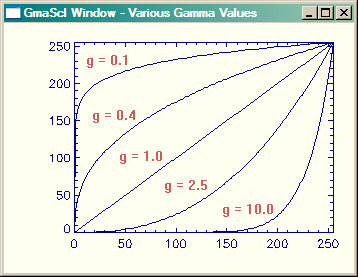#### cgLOGSCL

This is an image processing utility routine similar to cgGMASCL in that it scales input data into the range of 0 to 255. But the shape of the curve is fixed, unlike the shape in cgGmaScl, which is variable.
scaled = constant ( (1 + Double(image))

#### cgSDEVSCL

This is an image processing utility routine similar to BYTSCL, in that it scales input data into the range of 0 to 255. But it uses the standard deviation of pixels in the image to select upper and lower thresholds for the linear scaling. The standard deviation and mean are calculated for the image (excluding any value specified with the EXCLUDE keyword), then the standard deviation is multiplied by a factor (specified with the MULTIPLIER keyword), before being subtracted or added to the mean to select the lower and upper bounds for a linear scaling.

Here is an example of how the program can be used.

```   image = cgDemoData(5)
cgDisplay, 256*3, 256
!P.Multi = [0,3,1]
cgImage, image
cgImage, cgSDevScl(image)
cgImage, cgSDevScl(image, Exclude=0)
!P.Multi = 0
```

Here is the output.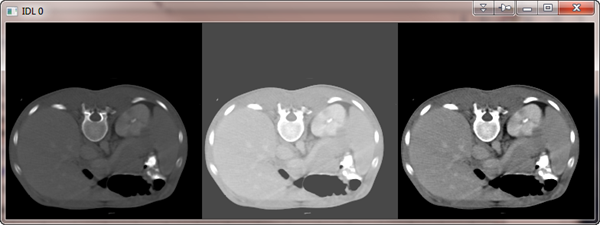#### cgSTRETCH

This widget program has been completely revised as a general purpose contrast enhancer for 2D images. It allows the user to interactively apply linear, gamma, log, or inverse hyperbolic sine stretches to an image. The user moves two threshold bars over the histogram plot of an image and the specified stretch is applied to the image data between the two threshold lines. For the log stretches, a third line shows the general shape of the scaling function. The program has been updated to allow both windows to be interactively resized, printed, as saved in a variety of file output types. The stretched image can be saved to the main IDL level. This program requires a number of programs from the Coyote Library, which you can download as a single zip file. The program was updated on 6 September 2007 to include four new stretches: (1) a linear 2 percent stretch, (2) a square root (log) stretch, (3) a histogram equalization stretch, and (4) a Gaussian normal stretch.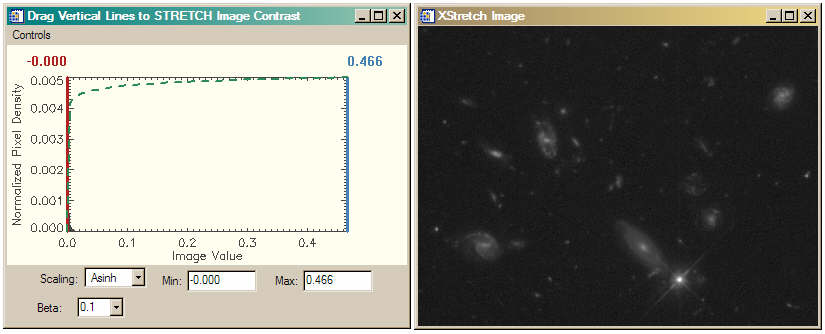#### CHECKERBOARD

This function returns a checkerboard image containing a user-specified number of alternating colored boxes (default of 8 as in a real checkerboard). The default image output size is 400 by 400, but can be specified by the user.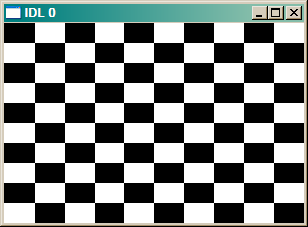#### FIT_ELLIPSE

The purpose of this utility routine is to fit an ellipse to a region of interest (or blob), which is defined by a one-dimensional array of pixel indices (such as is returned by the Where function. The method uses a "mass density" algorithm to calculate center of mass, orientation, major and minor axes, and semi-major and semi-minor axes of an ellise. The points that describe the ellipse are returned as a result of the function.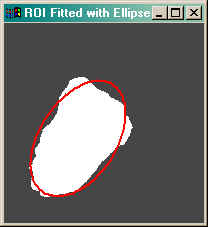#### HIST_ND

The purpose of this program is to calculate an N-dimensional histogram. Please don't ask me any questions about it. I didn't write it, and I'm not even totally sure I understand it. It was written by J.D. Smith and made available to the IDL community via the IDL newsgroup. I do know this: anything JD does is impressive. And the amount of code in this program (well, to be more exact, the lack of code in this program) is tremendously impressive. There is no one in the entire IDL community who understands histograms or vectorization better than JD.

#### HISTOMATCH

The purpose of this program is to perform "histogram matching" on an image. Histogram matching is a process whereby the image pixel distribution is manipulated to conform to the pixel distribution or histogram of another image. The final pixel distribution of the image will approximate that of the input histogram. For a more complete explanation of this process, see Histogram Matching.#### SCALEMODIS

It is not uncommon for true-color and false-color images created from MODIS reflectance channel data to appear dark and dingy. This IDL program, can be used to apply the same differential scaling the MODIS Rapid Response Team uses to produce the beautiful MODIS true-color images on its web page. In the figure below, the image on the left was produced with simple byte scaling, while the image on the right was produced by scaling with ScaleModis. See the article Bright MODIS Images for additional details.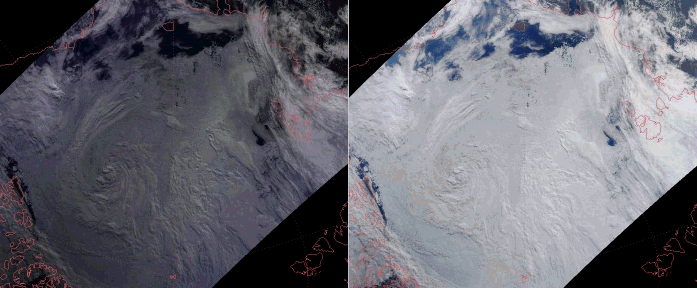#### SHARPEN

The purpose of this program is to sharpen an image with a Laplacian filter. Laplacian filters identify edges in images. In sharpening, these edges are added back to the image to produce a "sharpening" effect. For a more complete description of the method use, see the article Image Sharpening with a Laplacian Kernel.Last Updated: 27 March 2015